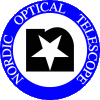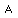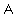# How to estimate S/N for ALFOSC polarimetry

High signal-to-noise ratios (S/N) are required for a good polarisation accuracy. Let Ep be the 1 sigma error in P (the degree of polarization). The S/N required to obtain a given accuracy Ep, assuming only photon shot noise, can be expressed as:

S/N(fo +fe) = sqrt(2) / (sqrt(n) * Ep)

where n is the number of half-wave-plate position angles, and S/N(fo +fe) is the signal to noise ratio of the flux of the combined components (ordinary + extraordinary) in one of the n intensity images, cf. Patat & Romaniello (2006, PASP 118, 146).

To better account for sky noise and readout noise through the use of our Exposure Time Calculator, however, the above formula is here modified to refer to the S/N of each dual-beam component. Assuming low polarization, fo is about equal to fe, and for half stellar intensity the relative sky noise is larger by a factor of 2 unless a focal plane mask is used. This may become important for faint targets. In the following we will thus refer to the signal to noise ratio of each dual-beam component as follows:

S/N = 1 / (sqrt(n) * Ep)

For linear polarimetry with ALFOSC/FAPOL with the default setup, n = 4, this means:

S/N = 0.5/ Ep

Example: to obtain an accuracy of ±0.3 % polarisation, i.e. Ep = 0.003, a signal to noise ratio of S/N = 167 is needed for each dual-beam component in each of the 4 images.

When using the Exposure Time Calculator for ALFOSC, one has to: 1) correct for the flux being split in two components by the calcite plate, and 2) correct for the flux losses by introducing the calcite and the 1/2 wave plate into the beam (check FAPOL commissioning report ). In practice, this means correcting the input magnitude of your target by adding the following magnitude offsets: 1.4 (U), 1.05 (B,V), and 1.0 (RI). The modified input magnitude is used in the Exposure Time Calculator to find the exposure time you need (for each of the n half-wave-plate positions) in order to reach the required S/N.

The uncertainty in the position angle (in degrees) goes as: 28.65 x Ep / P whenever P >> Ep.

The above estimation has some caveats:

• The limiting instrumental accuracy for FAPOL (lambda/2), as measured on bright standards, was found to be 0.06 % using 4 angles. It is possible to increase the accuracy using 8 or 16 angles. See Measured standard star accuracy.

• Note that the image quality is not undisturbed through the calcites, and a feature of the calcite is that both components can not be perfectly in focus at the same time. This means that one should not be too optimistic about the input FWHM in the ETC.

• at low degrees of polarization one has to take the non-gaussian distribution of the measurements into account, this will increase the effective errors.